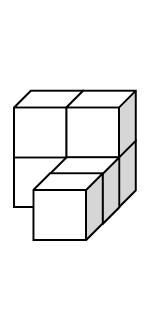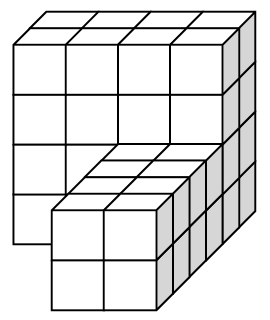### Home > GC > Chapter 9 > Lesson 9.1.5 > Problem9-48

9-48.

Consider the two similar solids below.Refresh your memory about linear scale factors by re-reading the Math Notes boxes in Lessons 8.2.1 and 9.1.5.

For help finding the surface area and volume of a solid, see the Math Notes box in Lesson 9.1.3.

1. What is the linear scale factor?

Find the linear scale factor by finding the zoom factor for any of the dimensions.

$2$

2. Find the surface area of each solid. What is the ratio of the surface areas? How is this ratio related to the linear scale factor?

The surface area can be found by counting or calculating the number of unit cube faces visible from all sides.

The surface areas are $24\text{ units}^2$ and $96\text{ units}^2$.
The ratio is $4$.
It is the square of the linear scale factor.

3. Now find the volumes of each solid. How are the volumes related? Compare this to the linear scale factor and record your observations.

The volumes can be found by counting the number of unit cubes in each solid or by finding the volume of the two rectangular prisms which make up each solid.

The volumes are $6\text{ units}^3$ and $48\text{ units}^3$.
The ratio is $8, 2^3$.

Use the eTool below to create the mat plan for part (a).
Click the link at right for the full version of the eTool: 9-48 HW eTool (Desmos)